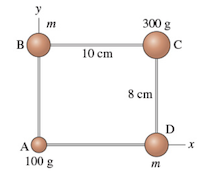# Problem: The four masses shown in the figure are connected by massless, rigid rods. Assume that m exttip{m}{m}= 210 g.a) What is the x-coordinate of the center of mass?b) What is the y-coordinate of the center of mass?c) Find the moment of inertia about an axis that passes through mass A and is perpendicular to the plane of the image.

###### Problem Details

The four masses shown in the figure are connected by massless, rigid rods. Assume that m = 210 g.
a) What is the x-coordinate of the center of mass?
b) What is the y-coordinate of the center of mass?
c) Find the moment of inertia about an axis that passes through mass A and is perpendicular to the plane of the image.